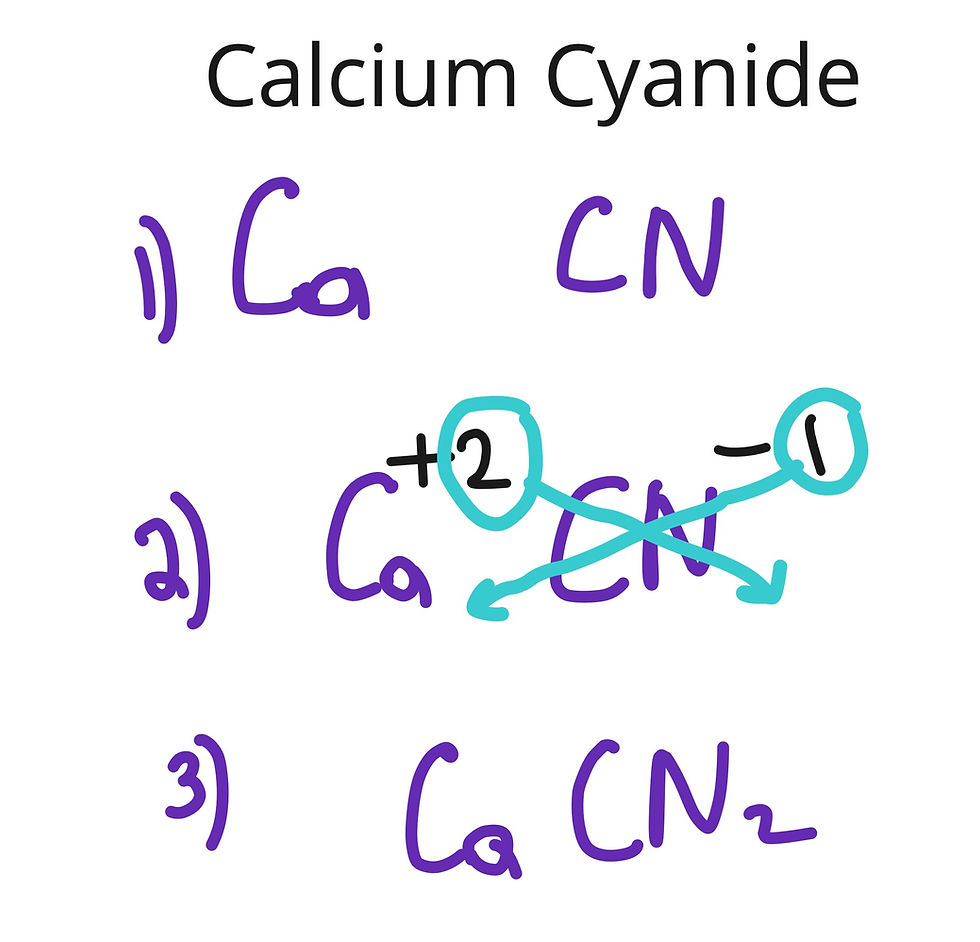top of page
Search

# The Complete Guide To Naming And Writing Formulas: Ionic, Molecular, Acids, Hydrates

Naming Ionic Compounds:

Before we begin, lets note a couple of things. Metals are to the left of the staircase on the Periodic Table. Nonmetals are to the right of the staircase on the Periodic Table.

Polyatomic ions are ions consisting of two or more elements. The names, formulas and charges of polyatomic ions are usually needed to be memorized for the test.

List of most common Polyatomic Ions:

There are several types of ionic compounds.

Metal + Nonmetal

Name of Metal + Name of Nonmetal ending in "ide"

Example: NaCl = sodium chloride

Metal + Polyatomic Ion

Name of Metal + Name of Polyatomic Ion

Example: Li2SO4 = lithium sulfate

Polyatomic ion + Nonmetal

Name of Polyatomic Ion + Name of Nonmetal ending in "ide"

Example: NH4Cl = ammonium chloride

Polyatomic ion + Polyatomic Ion

Name of the First Polyatomic Ion + Name of the Second Polyatomic Ion

Example: NH4OH = ammonium hydroxide

Ionic compounds with TRANSITION METALS

For metals that can have more than one possible charge, the name of the metal must be followed by a Roman numeral in parenthesis, indicating the charge.

Transition metals are located in the middle of the Periodic Table with atomic numbers 21 through 80.

How do we figure the charge of the transition metal?

1. Figure out the charge of the second ion. Group 16 = -2, Group 17= -1. The charges of polyatomic ions must be memorized. For example in the picture below we do not know the charge of iron but we know oxygen is -2 because it is in Group 16 on the Periodic Table.1. For ionic compounds, criss cross method is used for the formula. Figure out the charge of the transition metal by looking at the formula and charge of it's partner.

The charge of oxygen is -2. We see 2 next to iron. What did the charge of iron have to be to see 3 next to oxygen? It had to be 3.The name should be iron(III) oxide

If both partners have the same numbers like +2 and -2, they cancel to give 1 to 1 ratio. For example, the formula for iron(II) oxide would be FeO.

Let's do a little practice:

BaCl2

Barium chloride

BaSO4

CuO

Writing Formula for Ionic Compounds

1. Separate your compound into two parts and write their formulas.

2. Identify the charges of the two parts. If you have a Roman numeral, that's the charge of the metal. Group 1= +1 Group 2 = +2 Group 16 = -2, Group 17= -1 Al= +3. If something has a 1, we do not need to write the number 1.Practice:

barium sulfide

BaS

Aluminum Oxide

Iron(II)chloride

Naming Molecular Compounds

Molecular compounds consists of nonmetals only (usually).

We use prefixes to signify the number of atoms of each element

mono = 1

di = 2

tri = 3

tetra = 4

penta = 5

hexa = 6

hepta = 7

octa = 8

nona = 9

deca = 10

Name of nonmetal with prefix + name of nonmetal ending in "ide" with prefix

Note: We do NOT use prefix mono for the first element.

For example:

N2O4 = dinitrogen tetroxide

CO = carbon monoxide

Let's practice:

CO2

carbon dioxide

Cl2O7

NO2

SO3

Naming Hydrates

Hydrates are ionic compounds attached to a number of water molecules. An example of a hydrate looks like this: LiCl * 2H2O

Name the ionic compound + prefix (number of water molecules) hydrate

LiCl * 2H2O = lithium chloride dihydrate

CuSO4 * 5H2O = copper (I) sulfate pentahydrate

Practice:

LiBr * H2O

lithium monohydrate

Naming Binary Acids

Binary Acids consist of a hydrogen with a nonmetal in an aqueous solution

Hydro + name of nonmetal + ic acid

HBr (aq) = hydrobromic acid

H2S (aq) = hydrosulfuric acid

HCl (aq) = hydrochloric acid

Naming Oxoacids

Oxoacids consist of hydrogen + polyatomic ion that has an oxygen.

The name of the acid is based on the name of the polyatomic ion.

Polyatomic ion name Oxoacid name Example

per____ate ion per___ic acid H2SO5

___ate ion ___ic acid H2SO4

___ite ion ___ous acid H2SO3

hypo____ite ion hypo___ous acid H2SO2

As long as you remember the formula and charge for polyatomic ion ending in "ate", you can get the rest of the name as well. Let's say we have sulfate. Sulfate ion has 4 oxygens. Per____ate is one more oxygen than ate. So persulfate ion would have 5 oxygens. ___ite ion has one less oxygen than ate. Sulfite ion would have 3 oxygens. Hypo____ite ion has two less oxygens than ate. Hyposulfrous ion would have two oxygens.

Practice:

H3PO4

phosphoric acid

Chlorous Acid

Let's practice all the naming and formula writing now!

HI(aq)

hydroiodic acid

ammonium nitrite

hypochlorous acid

trisicilon tetranitride